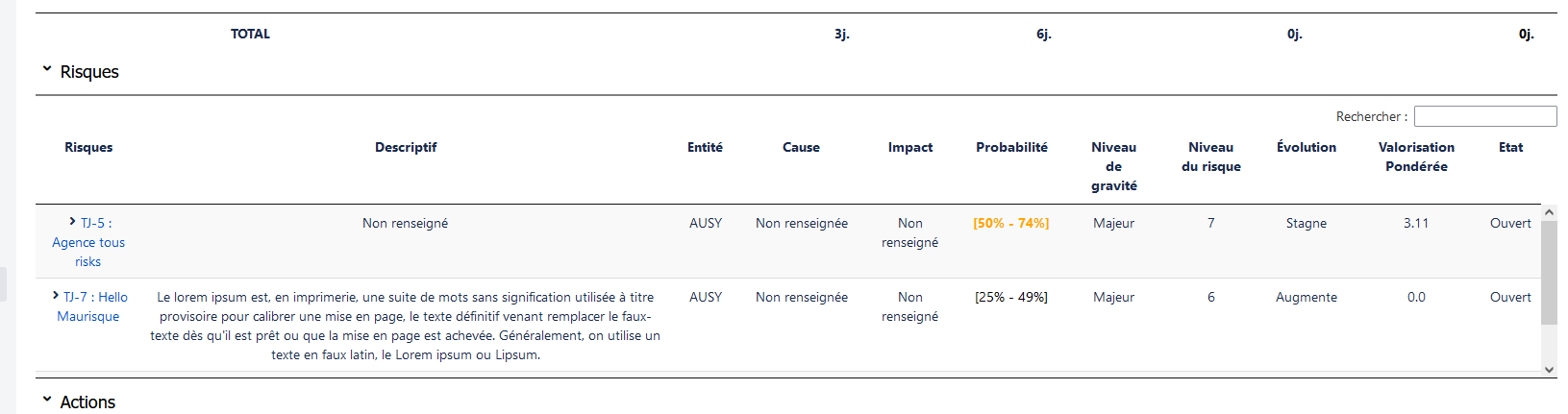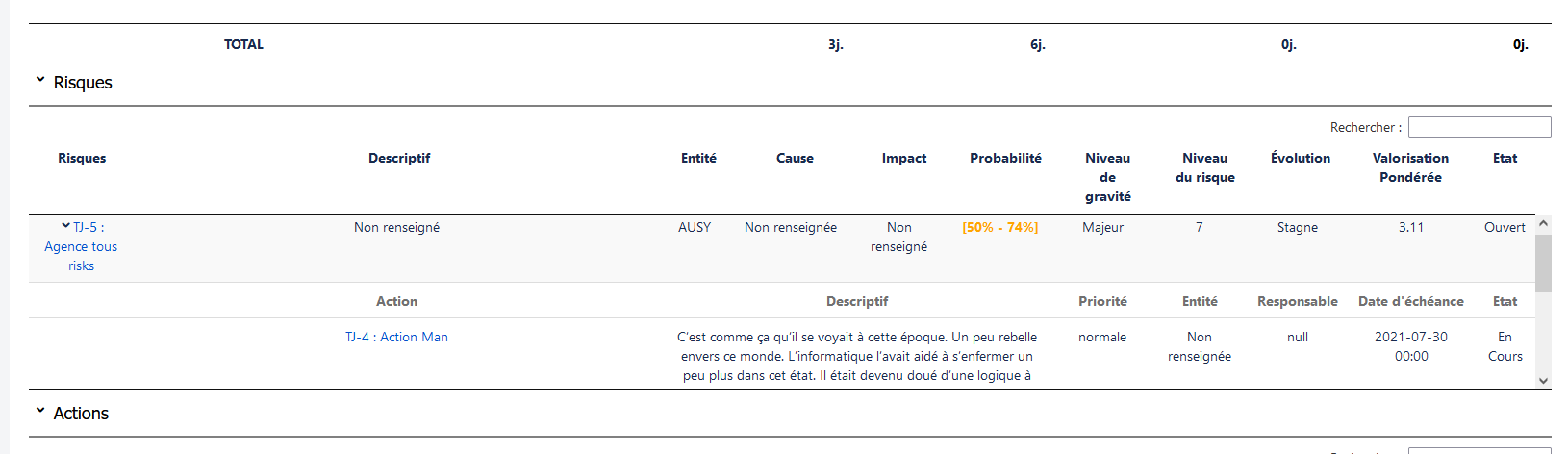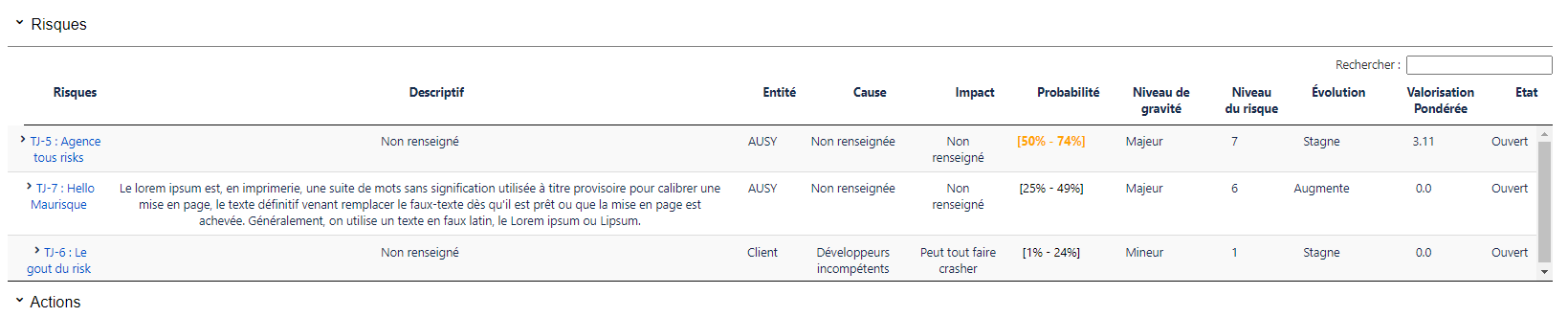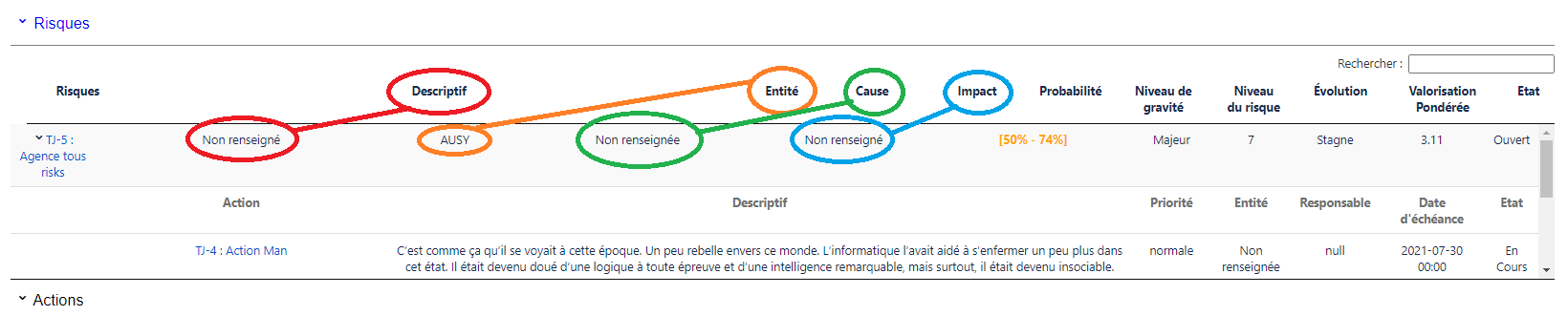# Datatables 1.10.4 - row not aligned anymore when expanded

Hey all,

I've a problem with using Datatables 1.10.4.
I work on a table of risk wich could contains another one if there is an Action linked to that risk. If there is a action, you can expand the row to show the sub table action.
When i try to expand my table using Firefox there is no problem. But when i do it using Chrome, all my row width's are modified and my table is broken.

Let me show you:

Firefox collapsed rowFirefox expandedEverything is ok, all rows/columns are inline as i wish.

This is what i get when i'm using Chrome:

Chrome collapsedChrome expanded (PROBLEM)More of 4 or 5 rows are not aligned as they were before i expand and show the sub row.

This is all the extensions i use with DataTablesAny help will be appreciated. Thanks

• edited July 21

Could be lots of things.

Are you using FixedHeader? Its not compatible with Datatables scrolling features like `scrollY` which it looks like you are using.

Do you have `style="width:100%"` defined on your `table` tag as shown in this example?

Can you post a link to your page or a test case showing the issues so we can help debug?
https://datatables.net/manual/tech-notes/10#How-to-provide-a-test-case

Kevin

• Here is my JS file:

``````function risks(col, direction, base, keyProj) {
var url = base + "/rest/ausy/reporting/latest/worklog/project/risks.json?project=" + keyProj;
var dataRow = {};
var index = 0;

\$('#risks_table').dataTable(
{
"processing": true,
"serverSide": false,
"scrollY": 180,
"info": false,
"paging": false,
"ajax": url,
"columnDefs": [
{
className: "center-top-cells",
"targets": [2, 3, 4, 5, 6, 7, 8, 9, 10, 11, 12]
},
{
"targets": [0, 1],
"visible": false,
"searchable": false
}
],
"order": [[col, direction]],
"columns": [
{"data": "key"},
{"data": "gravity"},
{"data": "name"},
{"data": "description"},
{"data": "responsibleEntity"},
{"data": "potentialCause"},
{"data": "projectImpact"},
{"data": "probability"},
{"data": "seriousness"},
{"data": "riskLevel"},
{"data": "evolution"},
{"data": "ponderedValuation"},
{"data": "state"},
],
"rowCallback": function (row, data) {
var feminineLabel = AJS.I18n.getText("unknown.feminine.label");
var masculineLabel = AJS.I18n.getText("unknown.masculine.label");
if (data !== undefined) {
\$('td:eq(0)', row).html("<a href='" + data.url + "' style='color : red'>" + data.key + " : " + data.name + "</a>");
}
else if (Number(data.riskLevel) >= 8 && data.linkedActions.length === 1) {
\$('td:eq(0)', row).html("<span id='" + data.key + "_iconRisk' class='aui-icon aui-icon-small aui-iconfont-collapsed' onclick=\"toggleRows('" + data.key + "')\"></span>" +
"<a href='" + data.url + "' style='color : red'>" + data.key + " : " + data.name + "</a>"
);
}
\$('td:eq(0)', row).html("<span id='" + data.key + "_iconRisk' class='aui-icon aui-icon-small aui-iconfont-collapsed' onclick=\"toggleRows('" + data.key + "')\"></span>" +
"<a href='" + data.url + "' >" + data.key + " : " + data.name + "</a>"
);
} else {
\$('td:eq(0)', row).html("<a href='" + data.url + "'>" + data.key + " : " + data.name + "</a>");
}
data.description === "null" ? \$('td:eq(1)', row).html(masculineLabel) : \$('td:eq(1)', row).html(data.description);
if (data.responsibleEntity.valueOf() === "null") {
\$('td:eq(2)', row).html(feminineLabel);
}
if (data.potentialCause.valueOf() === "null") {
\$('td:eq(3)', row).html(feminineLabel);
}
if (data.projectImpact.valueOf() === "null") {
\$('td:eq(4)', row).html(masculineLabel);
}
var color = data.evolution.valueOf() === "null" ? red : colorProbability(data.probability);
if (data.probability.valueOf() === "null") {
\$('td:eq(5)', row).html('<span style="color:' + color + '">' + feminineLabel + '</span>');
} else {
\$('td:eq(5)', row).html('<span style="color:' + color + '">' + data.probability + '</span>');
}
if (data.seriousness.valueOf() === "null") {
\$('td:eq(6)', row).html(masculineLabel);
}
if (data.riskLevel.valueOf() === "null") {
\$('td:eq(7)', row).html(masculineLabel);
} else {
\$('td:eq(7)', row).html(data.riskLevel.split('.'));
}
if (data.evolution.valueOf() === "null") {
\$('td:eq(8)', row).html(AJS.I18n.getText("risks.label.none"));
}
var ponderedValuation = \$('td:eq(9)', row);
data.ponderedValuation.valueOf() === "null" ? ponderedValuation.html(feminineLabel) : ponderedValuation.html(data.ponderedValuation);
if (data.state.valueOf() === "null") {
\$('td:eq(10)', row).html(masculineLabel);
}
index++;
}
},
language:
{
processing: AJS.I18n.getText("processing.label"),
search: AJS.I18n.getText("search.label"),
info: AJS.I18n.getText("info.label"),
infoEmpty: AJS.I18n.getText("infoEmpty.label"),
zeroRecords: "",
emptyTable: AJS.I18n.getText("risks.emptyTable.label"),
paginate:
{
first: AJS.I18n.getText("first.label"),
previous: AJS.I18n.getText("previous.label"),
next: AJS.I18n.getText("next.label"),
last: AJS.I18n.getText("last.label")
}
},
"initComplete": function (d) {
var indexNewRow = 0;
for (var i = 0; i < index; i++) {
if (dataRow[i].length > 0) {
\$('#risks_table > tbody > tr').eq(indexNewRow).after(
"<tr role='row' id='" + headerRowId + "' class='rowToHide'>" +
"<th></th>" +
"<th class='center-top-cells'>" + AJS.I18n.getText("action.label") + "</th>" +
"<th class='center-top-cells' colspan='4'>" + AJS.I18n.getText("description.label") + "</th>" +
"<th class='center-top-cells'>" + AJS.I18n.getText("priority.label") + "</th>" +
"<th class='center-top-cells'>" + AJS.I18n.getText("entity.label") + "</th>" +
"<th class='center-top-cells'>" + AJS.I18n.getText("leader.label") + "</th>" +
"<th class='center-top-cells'>" + AJS.I18n.getText("due-date.label") + "</th>" +
"<th class='center-top-cells'>" + AJS.I18n.getText("state.label") + "</th>" +
"</tr>"
);
indexNewRow++;
for (var j = 0; j < dataRow[i].length; j++) {
var subRowClass = d.json.data[i].key + '_row_actions';
"<tr role='row' class='" + subRowClass + "'>" +
"<td></td>" +
"<td class='center-top-cells'>" +
"<a href='" + dataRow[i][j].url + "'>" + dataRow[i][j].key + " : " + dataRow[i][j].name + "</a></td>" +
"<td class='center-top-cells' colspan='4'>" + dataRow[i][j].description + "</td>" +
"<td class='center-top-cells'>" + dataRow[i][j].priority + "</td>" +
"<td class='center-top-cells'>" + dataRow[i][j].responsibleEntity + "</td>" +
"<td class='center-top-cells'>" + dataRow[i][j].actionLeader + "</td>" +
"<td class='center-top-cells'>" + dataRow[i][j].due + "</td>" +
"<td class='center-top-cells'>" + dataRow[i][j].state + "</td>" +
"</tr>"
);
\$("." + subRowClass).hide();
indexNewRow++;
}
}
indexNewRow++;
}
}
});
}
``````